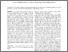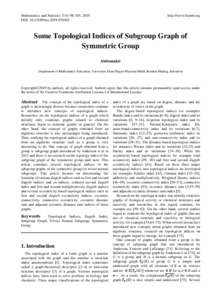Some Topological Indices of Subgroup Graph of Symmetric Group

Abdussakir, Abdussakir (2019) Some Topological Indices of Subgroup Graph of Symmetric Group. Mathematics and Statistics, 7 (4). pp. 98-105. ISSN 2332-2144Preview
Text (full text)
4638.pdf - Published Version

Full text available at: http://www.hrpub.org/journals/article_info.php?aid...

Abstract

The concept of the topological index of a graph is increasingly diverse because researchers continue to introduce new concepts of topological indices. Researches on the topological indices of a graph which initially only examines graphs related to chemical structures begin to examine graphs in general. On the other hand, the concept of graphs obtained from an algebraic structure is also increasingly being introduced. Thus, studying the topological indices of a graph obtained from an algebraic structure such as a group is very interesting to do. One concept of graph obtained from a group is subgroup graph introduced by Anderson et al in 2012 and there is no research on the topology index of the subgroup graph of the symmetric group until now. This article examines several topological indices of the subgroup graphs of the symmetric group for trivial normal subgroups. This article focuses on determining the formulae of various Zagreb indices such as first and second Zagreb indices and co-indices, reduced second Zagreb index and first and second multiplicatively Zagreb indices and several eccentricity-based topological indices such as first and second Zagreb eccentricity indices, eccentric connectivity, connective eccentricity, eccentric distance sum and adjacent eccentric distance sum indices of these graphs.

Item Type: Journal Article Topological Indices, Zagreb Index, Subgroup Graph, Trivial Normal Subgroup, Symmetric Group 01 MATHEMATICAL SCIENCES > 0101 Pure Mathematics > 010104 Combinatorics and Discrete Mathematics (excl. Physical Combinatorics) Faculty of Mathematics and Sciences > Department of Mathematics Abdussakir Abdussakir 03 Sep 2019 03:10View Item